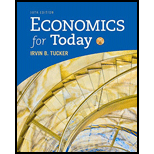# Key Concept: Income Distribution Which of the following most closely represents the share of total U.S. income to the poorest 20 percent of all U.S. families? a. Excessive resources devoted to producing a product b. 50 percent c. 25 percent d. 10 percent e. 5 percent### Economics For Today

10th Edition
Tucker
Publisher: Cengage Learning
ISBN: 9781337613040### Economics For Today

10th Edition
Tucker
Publisher: Cengage Learning
ISBN: 9781337613040

#### Solutions

Chapter P4, Problem 1KC
Textbook Problem

## Key Concept: Income DistributionWhich of the following most closely represents the share of total U.S. income to the poorest 20 percent of all U.S. families? a. Excessive resources devoted to producing a product b. 50 percent c. 25 percent d. 10 percent e. 5 percent

Expert Solution
To determine

The share of the total US income to the poorest 20 percent of all the US families.

Option 'e' is correct.

### Explanation of Solution

The economic growth is the increase in the inflation adjusted market value of goods and services in the economy over the time period. The distribution of income is the pattern in which the income is distributed within the population. Income inequality exists in an economy when a small percentage of population earns the largest share of national income, whereas the largest share of population earns the smallest share of national income.

Option (e):

The US economy is known as the capitalist economy and the country with the highest inequality. It is said that the poorest 20 percent of the population in the US earns only 5 percent of the national income of the US economy and that means option 'e' is correct.

Option (a):

The US economy depicts the highest level of income inequality where the highest share of income is earned by a smallest share of population and the highest share of population earns only a smallest share of national income. Thus, the excessive resources are not devoted to producing a product and hence, option 'a' is incorrect.

Option (b):

When 50 percent of income is earned by the poorest 20 percent of the population in the US economy, it indicates that there is a very low level of income inequality in the economy. Since the actual case is against it, option 'b' is incorrect.

Option (c):

When the poorest 25 percent of population has the share of 25 percent of the total income of the economy, it indicates that the level of income inequality is lower. However, the actual case in the US economy is such that the poorest 20 percent only earns the 5 percent of the US income and hence, option 'c' is incorrect.

Option (d):

The income distribution in the US economy is in such a way that only the 5 percent of the total US income is generated by the poorest 20 percent of the population. Thus, the given share of 10 percentage is higher than the actual share and thus, option 'd' is also incorrect.

Economics Concept Introduction

Income inequality:  Income inequality is the situation in the economy where a largest share of population earns only a smallest share of national income and vice versa.

### Want to see more full solutions like this?

Subscribe now to access step-by-step solutions to millions of textbook problems written by subject matter experts!

### Want to see more full solutions like this?

Subscribe now to access step-by-step solutions to millions of textbook problems written by subject matter experts!